ISSN: 2320-2459

All submissions of the EM system will be redirected to Online Manuscript Submission System. Authors are requested to submit articles directly to Online Manuscript Submission System of respective journal.

# A Construction Method and Application of Heart Sound Wavelet Neural Network

Cheng XF1*, Fu NT1, Yong MA2

1College of Electronic Science & Engineering, Nanjing University of Posts and Telecommunications, Nanjing, 210003 China.

2School of Computer Science, Nanjing University of Science and Technology, Nanjing, Jiangsu, 210094 China.

*Corresponding Author:
Cheng XF
College of Electronic Science & Engineering
Nanjing University of Posts and Telecommunications
Nanjing, 210003 China
Tel: 86-025-83482264
E-mail: [email protected]

Received Date: 15/10/2015; Accepted Date: 24/06/2016; Published Date: 26/06/2016

Visit for more related articles at Research & Reviews: Journal of Pure and Applied Physics

## Abstract

Constructing a targeted wavelet neural network is an effective method to enhance network performances and recognition effect. By introducing heart sounds wavelet of neural network hidden layer as the activation function, heart sound targeted learning and recognition technology are integrated deeply, to obtain a new heart sounds wavelet neural network. Selecting normal heart sounds and premature beats heart sound signals as the experimental objects, accuracy of recognition rate of heart sounds reached 97%. Compared with Morlet wavelet neural network and Mexicanhat wavelet neural network, the heart sound wavelet neural network shows obvious superiority on algorithm speed and convergence in heart sound recognition.

#### Keywords

Heart sound; Activate function; Heart sound wavelet neural network

#### Preface

Wavelet neural network is a wavelet-based neural network model, which combines time-frequency localization properties, focusing properties of wavelet transform and self-learning, adaptiveness, robustness, fault tolerance of neural networks together [1-3]. Wavelet neural network allows the network to avoid local optima fundamentally and accelerated the convergence speed, and has a strong learning and generalization capabilities. So, it has a wide range of applications in image processing, pattern recognition and other fields [4,5]. Constructing a targeted wavelet neural network is an effective method to improve recognition performance and network performance. Introducing targeted wavelet base to neural network hidden layer, specific learning and recognition of signals will reach to high degree of integration, in order to achieve targeted expression of feature extraction, classification and identification and solve classification problems under complex conditions. This has a very important significance on enhancing network performance and improving recognition results.

The principle of wavelet neural network constitution is that activation function of the neural network hidden layer node is replaced by wavelet function, and the corresponding weight of the input layer to the hidden layer is replaced by the scale expansion factor 'a' of the wavelet function, and the value of hidden layer to the output layer is replaced by the translation factor 'b', so the construction of wavelet neural network is actually the construction of activation function . At present, the "integration" of Wavelet and Neural Network has the following three ways:

(1) Using wavelet of continuous parameters as Implicit function of neural network;

(2) Using wavelet of multi-resolution as Implicit function of neural network;

(3) Using orthogonal basis functions as Implicit function of neural network.

Activation function can have non-linear factors and solve the problem which linear model can not. So the choice of activation function has a remarkable effect on the recognition rate or the convergence rate. The common activation functions include threshold type sub-stage function and sigmoid function . This article describes a method of construction of heart sound wavelet neural network, mainly analysing the model of heart sound wavelet neural network and configuration steps, giving its training algorithm. Specific application experiments and comparative analysis of heart sounds wavelet neural network proved that wavelet neural network has superiority on algorithm speed and convergence.

#### HS Wavelet Neural Network Structure

Figure 1 shows schematic diagram of heart sounds wavelet neural network recognition system, which is a method to enhance the effect of heart sound identification by restriction of targeted conditions.

Using heart sounds wavelet neural network recognition technology, on the one hand, the optimization techniques and physiological characteristics of heart sounds are combined together to get heart sound characteristic of physiological significance; on the other hand, take the heart sound optimization feature extraction and heart sound recognition integrated in a heart sounds classification network. In the hidden layer, taking heart sounds wavelet as the activation function, the heart sound recognition technology and targeted learning are in a high degree of integration, that is, using targeted levels oriented architecture of heart sounds wavelet neural network recognition system, it will achieve a targeted expression for heart sounds feature extraction and heart sounds classification to solve the problem of heart sound classification under complex conditions.

The steps of constructing the heart sounds wavelet neural network are as follow:

Step1: Structuring heart sounds wavelet base function

According to the configuration as shown in Figure 2 First, design wavelet scale filter function H(w), followed by the design of scale filter h(n) and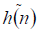, and then do scale space orthogonalization, finally wavelet base function are acquired [8,9].So that when the filter length is 10, vanishing moment is 5, a group of real solutions of heart sound wavelet base can be obtained: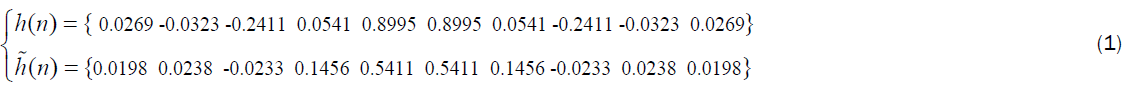According to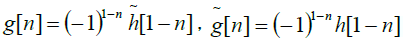the corresponding heart sounds wavelet filter g(n) and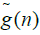would be: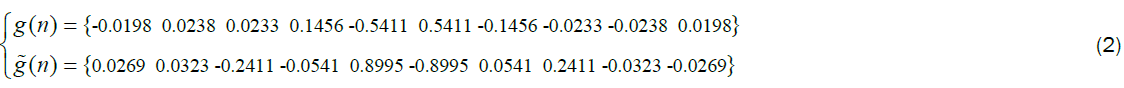Step2: Heart sounds wavelet coefficients time domain expression

The solution of Eq. (1) and (2) are substituted into two-scale equation (3):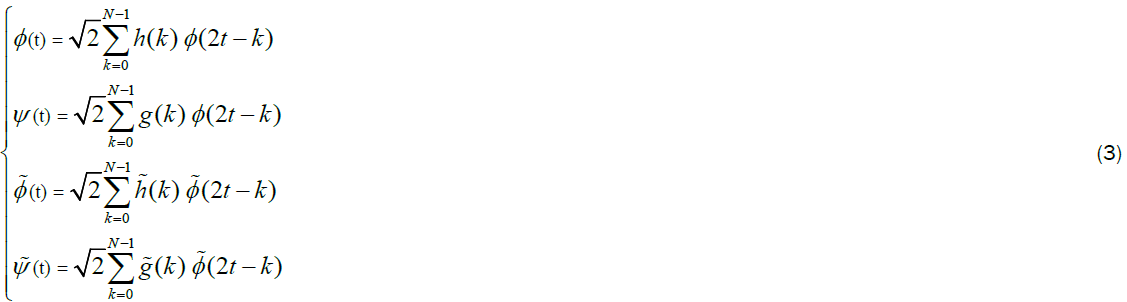So that when N=10, heart sounds wavelet base analysis in the form of time domain will be got, which is also a hidden layer function of the neural network.

Step 3: Replace activation function of neural network with heart sounds wavelet base function, and heart sounds wavelet neural network can be achieved:

Figure 3 wavelet neural network model structure diagrams:

Where, xi (i=1,2,….,m) is the i-th sample input of input layer, yk (k=1,2,….,n) is the k-th samples output of output layer, zi (z1,z2,…zf) is the wavelet function, the connection weight of input layer and hidden layer is w1ij; the connection weights of hidden layer and output layer is w2jk. So the input of the hidden layer neurons is: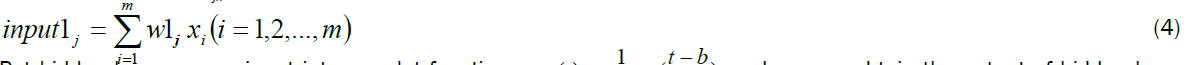Put hidden layer neuron input into wavelet function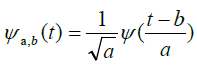and we can obtain the output of hidden layer neurons: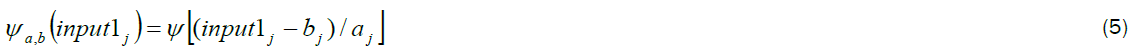From Eq. (4) and (5), the wavelet neural network model output can be expressed as: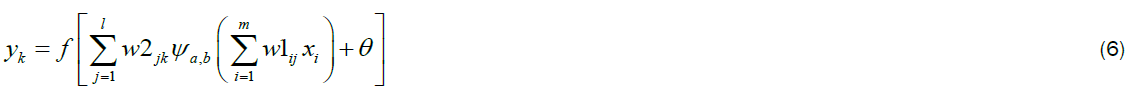Where θ represents the offset value, called the threshold value.

Normal heart sound signal model of a length of N can be described as: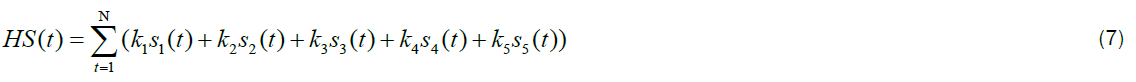Which, S1, S2 are the first and second heart sound signals respectively; S3, S4 are the third and fourth heart sound signals respectively, and its signal intensity is weak and generally not discussed [10,11]; S5 represents the murmur of heart sound; ki (I=1,2,3,4,5) is the combination coefficient.

Then take Eq. (3) and (7) into wavelet neural network model in Eq. (6), the heart sounds neural network can be constructed out. Its model structure is shown in Figure 4. The output of the heart sounds wavelet neural network is: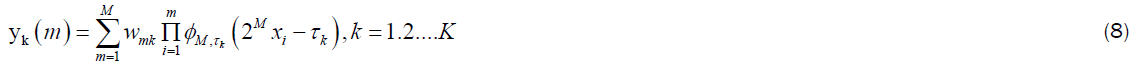#### Heart Sounds Wavelet Neural Network (HSWNN) Training Algorithm

The training of the neural network is actually to get the weights of the hidden layer to the output layer. In the heart sounds wavelet neural network (HSWNN), the sample dimension is relatively large; the cost of solving will become larger, therefore you can define a minimized error function in order to obtain the unique solution of weight wkp according to the characteristics of heart sounds.

The n-th samples of heart sound signals is HSn, we can get m eigenvalues from feature extraction where HSn=[hs1(n), hs2(n),……, hsm-1(n), hsm-1(n)], and its HSWNN training error is defined as follows:Where y is the expected output,is the actual output of the network, N is the total number of samples. So, HSWNN training algorithm is:Where: l is the number of iterations λ is the iterative step, gk (Xn) is the output of sample n in the N-th hidden layer nodes.

The heart sounds wavelet neural network minimized error training algorithm make error as a guide, continuously modifying parameters, in order to decrease error. Unlike the general search algorithm blind and unpredictable, tt has strong purpose, and the convergence rate clearly has advantages.

#### Applications of Heart Sounds Wavelet Neural Network

This paper selects premature beats and normal heart sound signals as experimental subjects, and the waveform of a group of normal heart sounds and premature beats heart sound signals are shown in Figure 5:

Figure 5: A group of normal heart sound waveforms and premature beats heart sound waveforms.

The two type’s heart sound signals shown in Figure 5 are decomposed into four layers respectively and the normalization energy values of the 16 frequency segments after the four decomposition are characterized as the feature vectors. Figure 6 shows the normalization energy diagrams of these two types of heart sound signals, where E9 is the sum of normalization energy values above 1000 Hz frequency band.

In this paper, in order to better evaluate the performance of wavelet neural network, we compare the usual Mexican hat wavelet neural networks and Morlet wavelet neural networks with it. Among them, waveform diagram of the self-configuring heart sounds, Mexican hat wavelet and Morlet wavelet base are shown as follows:

Our own built a heart sound database includes 323 groups of heart sounds Heart sounds wavelet is obtained by 30 samples normal heart sounds in the database. These heart sound samples are not included in the test sample of the experimental part. From the database, select 20 groups of normal heart sound signal and 20 groups of premature beats heart sound as the training data, and number them from Normal 1 to Normal 20, Beats 1 to Beats 20; then select 10 groups of premature beats heart sounds and 10 groups of normal heart sounds arbitrarily as the test data. Due to limited space, only two sets of data are listed.

In order to classify premature beats heart sound signals and normal heart sound signals, the recognition target values are divided into two categories: 100000000 is on behalf of premature beats heart sounds, and 010000000 represents normal heart sounds (Figure 7).

Firstly, the training data is inputted to heart sound wavelet neural network shown in Figure 3 for training, and we can adjust the network parameters according to the Eq. (9) and (10), and then take the test data into the trained wavelet heart sound neural network to validate, and finally obtain 20 output values. Compare the output value with the target value in the same circumstances. Figure 8 shows comparison charts of output value and target value among 10 groups of normal heart sound signal and 10 groups of premature beats heart sound signal.

From Figure 8, in the range of permitted error, using self-configuring heart sound wavelet neural network, 10 groups of normal heart sound signals and 10 groups of premature beats heart sound signal are detected, repeating 10 times, the average recognition rate reached to 97%. In the same test data, this paper repeated the above experiment with Morlet wavelet neural network and Mexican hat wavelet neural network, and obtained its error curve, operation time, and heart sound recognition rate. Error curve is shown in Figure 9, and the performance comparison results are shown in Table 1.

Analysis of Figure 9 can be learned, in heart sounds wavelet neural network, when the number of training reach 50 times, it has convergence, and Morlet wavelet neural network will not become convergence until 100 times, and Mexican hat wavelet neural network needs nearly 300 times before basic convergence; Tables 2 and 3 shows that, ensuring the correct identification rate unchanged, the heart sounds wavelet neural network computing cost is minimal. In summary, the heart sounds wavelet neural network has better convergence, less computation time and the rate of recognition reaches to 97%.

Compute time Convergence Recognition rate
HS wavelet neural network 0.0156 Fast 97%
Mrolet wavelet neural network 0.0312 Amid 97%
Mexican hat wavelet neural network 0.0312 Slow 97%

Table 1: Performance comparison of three wavelet neural network.

Group E1 E2 E3 E4 E5 E6 E7 E8 E9
Beat 1 0.0554 0.3038 0.2091 2.1581 0.0412 0.2071 0.8093 3.2430 1.2542
Beat 2 0.0374 0.1314 0.1667 0.7537 0.0209 0.1911 1.3794 3.4995 1.1320
Normal 1 59.236 4.0632 0.0167 0.5612 0.0034 0.0035 0.0172 0.0385 0.0144
Normal 2 51.867 7.4678 0.0300 1.5634 0.0004 0.0062 0.0282 0.0279 0.0092

Table 2: Energy eigenvalue of the training data.

Group E1 E2 E3 E4 E5 E6 E7 E8 E9
Beat 1 0.0288 0.3545 0.3633 2.2519 0.2922 1.0104 1.6630 12.174 6.1445
Beat 2 0.0114 0.3676 0.0833 0.8241 0.0290 0.2026 0.5465 1.3602 1.1216
Normal 1 42.524 0.0002 0.0005 0.0001 0.0001 0.0004 0.0003 0.0005 0.0001
Normal 2 5.1044 3.8369 0.0137 0.0487 0.0002 0.0029 0.0232 0.0252 0.0011

Table 3: Energy eigenvalue of the test data.

#### Conclusion

This paper mainly completed the following work:

1. A detailed description of the method of the heart sounds wavelet replacing the activation function. Give heart sound wavelet neural networks structure steps;

2. According to the characteristics of heart sounds, constructing wavelet neural network and its training algorithm, we obtained a wavelet neural network, which has more advantages in the processing of heart sounds, providing an effective method for engineering application of wavelet neural network;

3. Selecting the normal heart and premature beats heart sound signals to do simulation experiment, the experimental results show that the correct rate of heart sounds wavelet neural network reached 97%, and in terms of convergence and algorithm speed, heart sounds wavelet neural network far exceeds the Morlet wavelet neural network and Mexican hat wavelet neural network in heart sound recognition.

#### Acknowledgement

*Project supported by the National Natural Science Foundation of China (Grant No.61271334) “new method of Research and application on the heart sound features extraction for identification”.